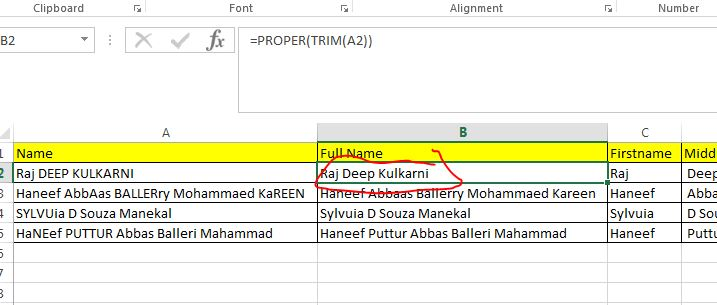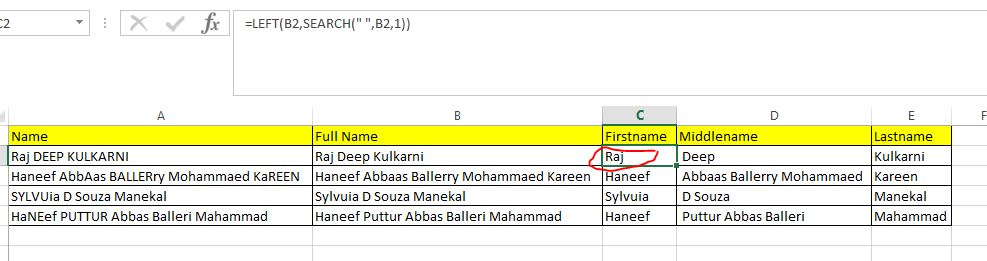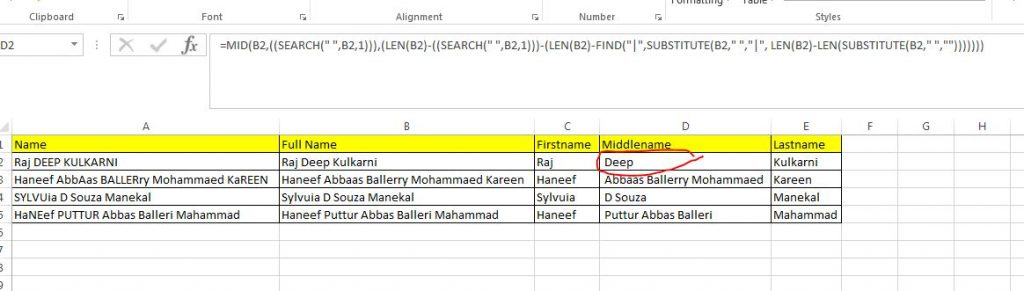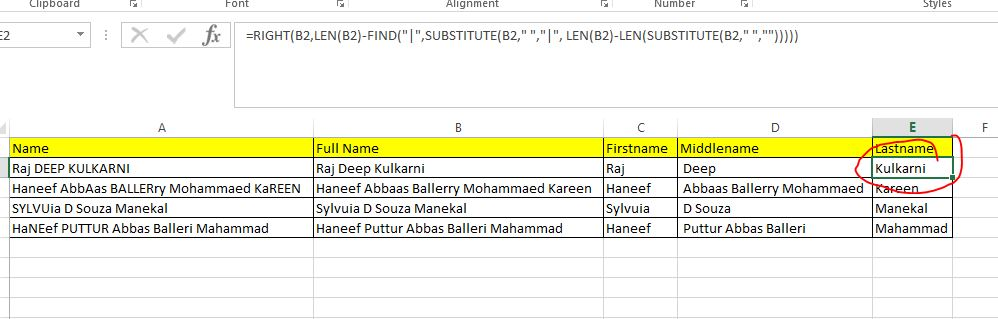# How to Clean up names and split names in Excel as First , Middle and Last Name

By | June 26, 2016

Scenario :

You have a excel file with one column which is full name. It needs to clean up by trimming extra spaces, convert into sentense case and finally split into three names .

Example :

Orginal Name : MAHAMMAD HanEEF BALLeri PUttur    = > Firstname : Mahammad  Middlename : Haneef Balleri  Lastname : Puttur

Steps

• Put the Row Names in Column A
• Column B , put a formula to trim and also to convert to Sentence Case  B2 =PROPER(TRIM(A2))
• column C , put a formula to extract the first name from B2 .  C2=LEFT(B2,SEARCH(” “,B2,1))
• column D , Put a formula to extract the Middle Name from B2 .   D2 = MID(B2,((SEARCH(” “,B2,1))),(LEN(B2)-((SEARCH(” “,B2,1)))-(LEN(B2)-FIND(“|”,SUBSTITUTE(B2,” “,”|”, LEN(B2)-LEN(SUBSTITUTE(B2,” “,””)))))))
• column E, Put a formula to extract the Last Name from B2,  E2 = RIGHT(B2,LEN(B2)-FIND(“|”,SUBSTITUTE(B2,” “,”|”, LEN(B2)-LEN(SUBSTITUTE(B2,” “,””)))))Splitting Full Name into First , Middle and Last name in Oracle Pl/SQL

If you wish to achieve the same in oracle use the following trick>

–Firstname

select substr (‘Mohammed Hannef Marakkar Puttur’,1,instr(‘Mohammed Hannef Marakkar Puttur’,’ ‘)) from dual;

— Last Name

select substr (‘Mohammed Hannef Marakkar Puttur’,instr(‘Mohammed Hannef Marakkar Puttur’,’ ‘,-1)) from dual;

–Middle Name

select substr(substr (‘Mohammed Hannef Marakkar Puttur’,1,instr(‘Mohammed Hannef Marakkar Puttur’,’ ‘,-1)),instr(‘Mohammed Hannef Marakkar Puttur’,’ ‘)) from dual